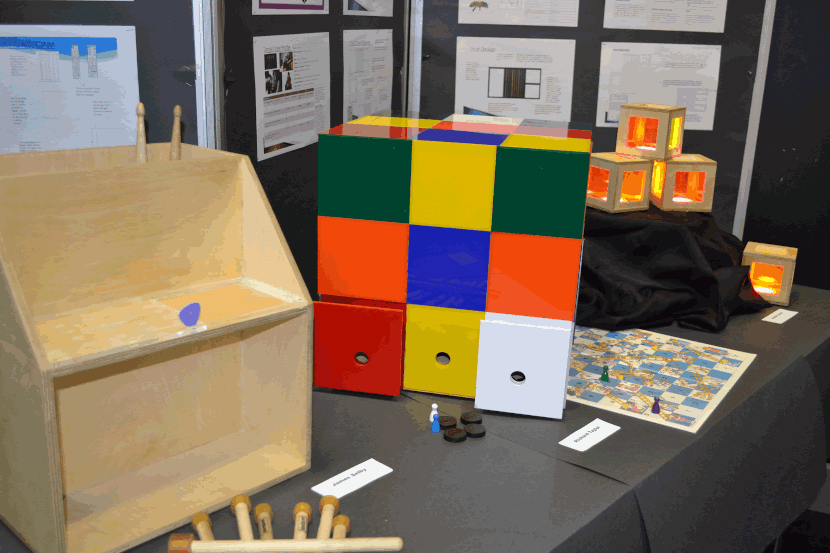## Mathematics

Exam Board

Edexcel/Pearson

##### Contact

A-Level Mathematics is widely recognised as an excellent qualification to have, both by universities for many courses, and also employers - it is a subject which can open doors as it is viewed as a subject which demonstrates that you are intelligent and have strong problem-solving and reasoning skills.

##### Year 12 Topics

Pure Maths
1 Algebraic Expressions
3 Equations and Inequalities
4 Graphs and Transformations
5 Straight Line Graphs
6 Circles
7 Algebraic Methods
8 Binomial Expansions
9 Trigonometric Ratios
10 Trigonometric Identities and Equations
11 Vectors
12 Differentiation
13 Integration

Applied Maths
S1 Data Collection
S2 Measures of Location and Spread
S3 Representations of Data
S4 Correlation
S5 Probability
S6 Statistical Distributions
S7 Hypothesis Testing
M8 Modelling
M9 Constant Acceleration
M10 Forces and Motion
M11 Variable Acceleration

##### Year 13 Topics

Pure Maths
1 Algebraic Methods
2 Functions and Graphs
3 Sequences and Series
4 Binomial Expansions
6 Trigonometric Functions
7 Trigonometry and Modelling
8 Parametric Equations
9 Differentiation
10 Numerical Methods
11 Integration
12 Vectors

Applied Maths
S1 Regression and Correlation
S2 Probability
S3 The Normal Distribution
M4 Moments
M5 Forces and Friction
M6 Projectiles
M7 Application of Forces
M8 Further Kinematics

##### Exams

You will have 3 exam papers at the end of Year 13.
- Paper 1 and 2 are Pure Mathematics (and makes up 2/3 of the marks)
- Paper 3 is Statistics and Mechanics (and is worth 1/3 of the marks)

There is no specific content for Paper 1 and 2, and each paper is worth 1/3 of the total qualification.